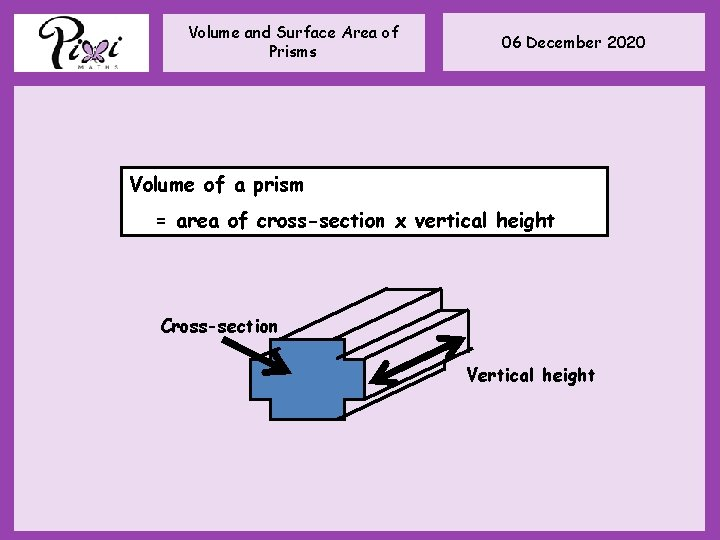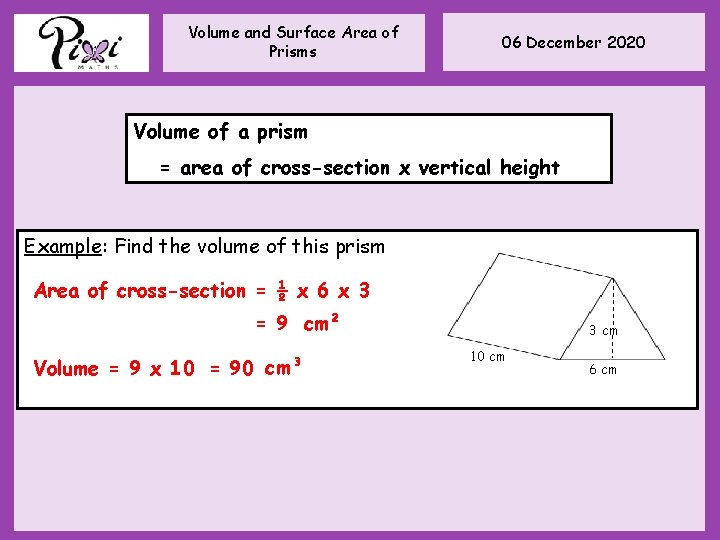# Volume and Surface Area of Prisms 06 December

• Slides: 7Volume and Surface Area of Prisms 06 December 2020 Cross Section – The shape of the slice Prism – A shape that has the same cross section all the way throughVolume and Surface Area of Prisms 06 December 2020 A prism is a 3 -D shape which has the same cross-section throughout its height. Cuboid Triangular prism Pentagonal prismVolume and Surface Area of Prisms 06 December 2020 Volume of a prism = area of cross-section x vertical height Cross-section Vertical heightVolume and Surface Area of Prisms 06 December 2020 Volume of a prism = area of cross-section x vertical height Example: Find the volume of this prism 25 x 7 = 175 cm³ 25 cm 2 7 cmVolume and Surface Area of Prisms 06 December 2020 Volume of a prism = area of cross-section x vertical height Example: Find the volume of this prism Area of cross-section = ½ x 6 x 3 = 9 cm² Volume = 9 x 10 = 90 cm³ 3 cm 10 cm 6 cmVolume and Surface Area of Prisms 06 December 2020 Volume of a prism = area of cross section x length Calculate the volume of this cylinder. Give your answer to 1 d. p. Area of cross-section = π x 12² = 452. 389… cm² Volume = 452. 389… x 20 = 9047. 8 cm³ Calculate the volume of this cylinder. Give your answer to 3 s. f. Area of cross-section = π x 5² = 78. 539… cm² Volume = 78. 539… x 12 = 943 cm³Volume and Surface Area of Prisms 06 December 2020 Assess Time! How confident do you feel about this topic? Blue, Yellow or Green!